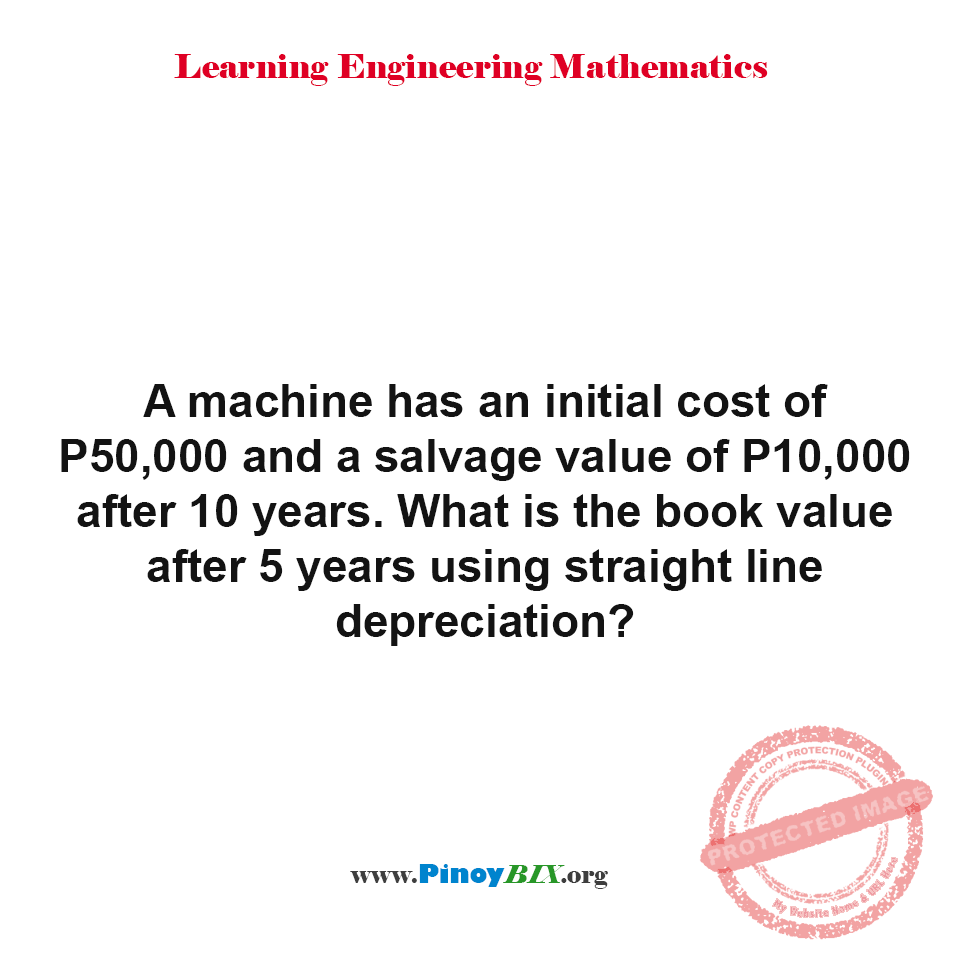# Solution: What is the book value after 5 years using straight line depreciation?

(Last Updated On: January 9, 2020)#### Problem Statement: EE Board April 1997

A machine has an initial cost of P50,000 and a salvage value of P10,000 after 10 years. What is the book value after 5 years using straight line depreciation?

• A. P30,000.00
• B. P31,000.00
• C. P30,500.00
• D. P31,500.00

The book value after 5 years using straight line depreciation is P30,000.00.

Solution:

### Latest Problem Solving in Engineering Economics (Annuity, Depreciation, Bonds, Breakeven, etc.)

More Questions in: Engineering Economics (Annuity, Depreciation, Bonds, Breakeven, etc.)

#### Online Questions and Answers in Engineering Economics (Annuity, Depreciation, Bonds, Breakeven, etc.)

PinoyBIX Engineering. © 2014-2021 All Rights Reserved | How to Donate? |